The NCERT Solution for Class 11 Chemistry includes answers to every question from the NCERT text book’s exercise. Top students love SWC NCERT Solutions because they are very effective. In general, Class 11 is regarded as the most significant year in a student’s professional development. The NCERT answers for class 11 Chemistry were created with the goal of providing students with the most help possible.

Answers to the class 11 Chemistry questions provided in the exercise might be challenging for students for a number of reasons. One should not omit any NCERT textbook content in order to get the highest possible grade. Use the Swastik Classes’ NCERT answers for Chemistry class 11 as a resource. Important exam-based questions are covered in depth in each chapter.

## NCERT SOLUTIONS FOR CLASS 11 CHEMISTRY CHAPTER 9- HYDROGEN – Exercises

### Chapter-9 Hydrogen

1. Explain on the general basis of electronic configuration – the actual position of hydrogen in periodic table.

The 1st element in the periodic table is hydrogen. Hydrogen exhibits dual behaviour because it has only 1 electron on its one ‘S’ shell.(i.e.,) hydrogen resembles both halogens and alkali metals.

Electronic configuration of hydrogen = [1s1]

Hydrogens resemblance with alkali metals:

Hydrogen has 1 valence electron on its valency shell like alkali metals.

[He] 2s – Li

1s1 – H

[Ne] 3s1 – Na

Therefore, to form a uni positive ion, it can lose one of its electron.

To form halides, oxides and sulphides, it combines with electro –ve elements which is same as alkali metals.

Hydrogens resemblance with halogens:

Only 1 electron is required to complete their respective octets for both the halogen and hydrogen.

H : 1s 1

F : 1s 2 2s 2 2p 5

Cl : 1s 2 2s 3 2p 6 3s 2 3p 5

It forms several covalent compounds and diatomic molecule like halogens. Even though hydrogen has certain similarity among both halogen and alkali metal, it differs from them. Hydrogen wont possess metallic characteristics; it possesses higher ionization enthalpy and reacts less than halogens.

Due to these reasons, hydrogen cant be replaced with alkali metal of 1st group or with the halogens of 2nd group. Therefore, it is best to place hydrogen separate in the periodic table.

2. Mention the names of isotopes of hydrogen and mass ratio of  isotopes.
3 isotopes:
(i)  tritium   31H or T
(ii)  protium 31H
(iii) deuterium 21H or D
Mass Ratio: Tritium + Protium + deuterium = 1 : 2 : 3

3. Hydrogen occurs in diatomic form rather than monoatomic form under certain normal conditions. Explain why?
Answer: The ionization enthalpy of hydrogen atom is very high (1312 kJ mol–1). Hence, it is very hard to remove its only electron. As a result, its tendency to exist in the monoatomic form is rather low. Instead, hydrogen forms a covalent bond with another hydrogen atom and exists as a diatomic (H2) molecule.

4. Explain how the production of di hydrogen which is obtained from the process ‘coal gasification’ can be increased?
By the process of coal gasification, di hydrogen is produced as,

C(g) + H2O(g) → CO(g) + H2(g) [C – Coal ]

Reaction with carbon monoxide with steam in the presence of a catalyst (iron chromate) results in increase in the yield of di hydrogen.
CO(g) + H2O(g) → CO2(g) + H2(g)

The above reaction is known as water- gas shift reaction. The carbon dioxide can be removed by scrubbing it with sodium arsenite solution .

5. Explain by electrolytic method – the bulk preparation of dihydrogen and the role of electrolyte in the process.
Answer: The preparation of di hydrogen is by the electrolysis of acidified or alkaline water using platinum electrodes. Generally, 15 – 20% of an acid (H2 SO 4) or a base (NaOH) is used.

At the cathode, reduction of water occurs as:
2H2O+2e → 2H2 + OH

At the anode, oxidation of OH– ions takes place as:
2OH → H2O + 1/2O2 + 2e

Net reaction is represented as
H2O(1) → H2(g) + 1/2O2(g)

Due to the absence of ions, the electrical conductivity of pure water is too low. Hence, electrolysis of pure water takes place at a low rate. The rate of electrolysis increases if an electrolyte such as an base or acid is added to the process. The electrolyte is added which makes the ions available in the process for the conduction of electricity and for electrolysis to take place.

6. Complete the following reactions:
(i)  H2(g) + Mm O0(g) Δ
(ii)  CO(g) + H2(g)
(iii)  C3H8(g) + 3H2O(g) →
(iv) Zn(g) + NaOH(aq) heat/heat  →

(i)H2(g) + Mm O0(g) → mM(s) + H2O(l)

(ii)CO(g) + H2(g) → CH3OH(l)

(iii)C3H8(g) + 3H2O(g) → 3CO(g) + 7H2(g)

(iv)Zn(g) + NaOH(aq) → Na2 ZnO2(aq) + H2(g)

7. Discuss in terms of chemical reactivity of di hydrogen – the consequences of high enthalpy of H–H bond.

Answer: The ionization enthalpy of H–H bond is higher (1312 kJ mol–1 ) which shows  that hydrogen has a low tendency to form H+ ions. Its ionization enthalpy value is comparable to that of halogens. Hence, it forms
→ a large number of covalent bonds,
→ diatomic molecules (H2),
→ hydrides with element.
Hydrogen does not possess metallic characteristics (lustre, ductility, etc.) like metals because ionization enthalpy is very high.

8. What do you understand by?
(i) electron rich – compounds of hydrogen
(ii) electron-precise, and
(iii) electron-deficient.
Provide justification with suitable examples.

Molecular hydride is classified on the basis of the presence of the bonds and total number of electrons in their Lewis structures as:
1.Electron-deficient hydrides
2.Electron-precise hydrides
3.Electron-rich hydrides

An electron-deficient hydride has very less electrons, less than that required for representing its conventional Lewis structure.

E.g. diborane (B2 H6)
In B2 H6, there are 6 bonds in all, out of which only 4 bonds are regular 2 centered-2 electron bonds.
The remaining 2 bonds are 3 centered-2 electron bonds i.e., 2 electrons are shared by 3 atoms. Hence, its conventional Lewis structure cannot be drawn.
By conventional Lewis structure, an electron-precise hydride has a sufficient number of electrons to be represented.

E.g. CH4
The Lewis structure can be written as: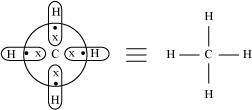4 regular bonds are formed where 2 electrons are shared by 2 atoms. An electron-rich hydride contains excess electrons as lone pairs.

E.g. NH3
There are 3 regular bonds in all with a lone pair of electrons on the nitrogen atom.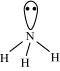9. Mention the characteristics from an electron-deficient hydride with respect to its chemical reactions and structure?
Answer: To form a regular bond, an electron-deficient hydride does not have sufficient electrons in which 2 electrons are shared by 2 atoms.
e.g., B2 H6, Al2 H6 etc.
Conventional Lewis structures cannot able to represent these hydrides. B2H6, for example, contains 4 regular bonds and 2 3centered-2 electron bond. Its structure can be represented as:

They have a tendency to accept electrons because, these hydrides are electron-deficient. Hence, they act as Lewis acids.
B2H+ 2NMe → 2BH3.NMe3

B2H6 + 2CO → 2BH3. CO

10. Do you expect the carbon hydrides of the type (Cn H2n + 2 ) to act as ‘Lewis’ base or acid? Justify.
For carbon hydrides which belong to type (Cn H2n + 2), the following hydrides are possible for
n = 1⇒CH4
n = 2 ⇒C2 H6
n = 3⇒C3 H

For a hydride to act as a Lewis acid, it should be electron deficient.
Lewis acid = electron accepting
Also, for a hydride to act as a Lewis base, it should be electron rich.
Lewis base = electron donating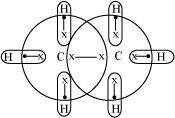Taking C2 H6 as an example, the total number of electrons are 14  and the total covalent bonds are 7. Hence, the bonds are regular 2e -2centered bonds.
Hence, hydride C2 H6 has sufficient electrons to be represented by a conventional Lewis structure. Therefore, it is an electron-precise hydride, having all atoms with octets. Thus, it can neither accept nor donate electrons to act as a Lewis base or Lewis acid.

11. What is “non-stoichiometric hydrides”? Is it possible for the formation of alkali metals by this type? Explain.
Answer: Non-Stoichiometric hydrides are hydrogen-deficient compounds which is formed by the reaction of dihydrogen with d- block and f- block elements. These hydrides do not follow the law of constant composition.

Eg:
LaH2.87,
YbH2.55,
TiH 1.5 – 1.8  etc.
Alkali metals form stoichiometric hydrides which are naturally ionic. Hydride ions have comparable sizes (208 pm) with alkali metal ion. This results in strong binding force between the constituting metal and hydroxide ion. As a result, stoichiometric hydrides are formed.
Alkali metals will not form non-stoichiometric hydrides.

12. What are the ways that the metallic hydrides is useful for hydrogen storage?
Answer: Metallic hydrides are hydrogen deficient. They don’t follow the law of constant composition.
It has been established that in the hydrides of Pd, Ac, Ni, and Ce, hydrogen occupies the interstitial position in lattices which allow further absorption of hydrogen on these metals.
Metals like Pt and Pd have the capacity to accommodate a large volume of hydrogen. Hence, metallic hydrides serve as a source of energy and used for the storage of hydrogen.

13. Explain the function of atomic hydrogen torch in welding and cutting purposes.
Answer: The atomic hydrogen torch is also known as oxy- hydrogen torch. These atoms are produced through dihydrogen dissociation with the help of an electric arc which results in huge amount of energy.
Energy released = 435.88 kJ mol-1
This energy is used in generation of 4000 K temperature which is used in cutting and welding of metals.
Therefore atomic hydrogen torches are used for this purpose i.e., it allows to recombine on the particular surface to be welded for the generation of particular temperature.

14. Among HF, NH3, and H2O, which has the highest magnitude of hydrogen bonding. Explain why?
The extent of hydrogen bonding mainly depends on
(i) Electronegativity
(ii) Number of hydrogen atoms available for bonding.
Among oxygen, fluorine and nitrogen, the increasing order of their electro negativities are N < O < F.
Therefore, the expected order of the extent of hydrogen bonding is HF > H2O > NH3.
But, the actual order is H2O > HF > NH3.
Even though fluorine is more electronegative than oxygen, the extent of hydrogen bonding is high in water.
There is a shortage of hydrogens in HF, whereas there are exactly the right numbers of hydrogens in water. As a result, only straight chain bonding takes place.
On the other hand, oxygen forms a huge ring-like structure through its high ability of hydrogen bonding.
The extent of hydrogen bonding is limited In case of ammonia, because nitrogen has only 1 lone pair. Therefore, it cannot satisfy all hydrogens.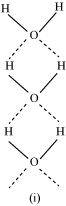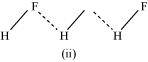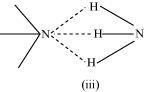15. When saline hydrides reacts violently with water, it produces fire. Can a familiar fire extinguisher CO2 be used in this scenario?
Saline hydrides [i.e.,LiH, NaH etc.] react with water to form hydrogen gas
And a base. The chemical equation to represent this reaction is
MH(g) + H2O(aq) → MOH(aq) + H2(g)
This reaction behaves violent and also fire is produced from this.
Dioxygen weights lighter than CO2. CO2 is commonly used as fire extinguisher as it covers the fire like blanket and inhibits the dioxygen supply, thereby dousing the fire.
It can be used in this scenario also. It weights higher than di hydrogen and effective in isolating the burning surface from dioxygen and dihydrogen.

16. Arrange the following
(i) LiH, NaH and CsH in order of increasing ionic character.
(ii) CaH2, BeH2 and TiH2 in order of increasing electrical conductance.
(iii) NaH, MgH2 and H2O in order of increasing reducing property.
(iv). H–H, D–D and F–F in order of increasing bond dissociation enthalpy.

(i) The electrical conductance of a molecule mainly depends on its covalent or ionic nature. CaH2 is an ionic hydride, which conducts electricity in the molten state. Titanium hydride, TiH2 is metallic in nature and conducts electricity at room temperature.  Covalent compounds do not conduct, whereas Ionic compounds conduct.  BeH2 is a covalent hydride. Hence, it does not conduct.
Hence, the increasing order of electrical conductance is as follows:
BeH2 < CaH2 < TiH2

(ii) The ionic character of a bond is dependent on the electro negativities of the atoms involved. The higher the difference between the electro negativities of atoms, the smaller is the ionic character. Electronegativity decreases down the group from Lithium to Caesium. Hence, the ionic character of their hydrides will increase (as shown below).
LiH < NaH < CsH

(iii) The bond pair in D–D bond is more strongly attracted by the nucleus than the bond pair in H–H bond. This is because of the higher nuclear mass of D2. The stronger the attraction the greater will be the bond strength and the higher is the bond dissociation enthalpy.
Bond dissociation energy depends upon the bond strength of a molecule, which in turn depends upon the repulsive and attractive forces present in a molecule.
Hence, the bond dissociation enthalpy of D – D is higher than H – H. However, bond dissociation enthalpy is the minimum in the case of F–F. The bond pair experiences strong repulsion from the lone pairs present on each F-centre.
Therefore, the increasing order of bond dissociation enthalpy is as follows:
F–F < H–H < D–D

(iv)  Ionic hydrides are strong reducing agents.  NaH can easily donate its electrons. Hence, it is most reducing in nature. Both, MgH2 and H2O are covalent hydrides. H2O is less reducing than MgH2 since the bond dissociation energy of H2O is higher than MgH2. Hence, the increasing order of the reducing property is H2 O < MgH2 < NaH.

17. Compare the structures of H2 O2 and H2O.
Answer: The water molecule will be displayed with a bond angle of 104 .5  has a bent form in gaseous phase. The O-H bond length is 95.7 pm.
Structure : Hydrogen peroxide has a non- planar structure both in solid and gas phase.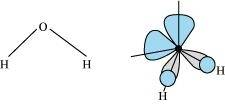The dihedral angle in gas and solid phase is 90.2o and 111.5o.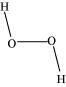18. Define ‘auto – protolysis’ of water and its significance.
Auto-protolysis (self-ionization) of water is a chemical reaction in which 2 water molecules react to produce a hydroxide ion (OH–) and a hydronium ion (H3O+).
The reaction involved can be represented as:
H2O(l) + H2O(l) ↔ H3O + (aq) + OH(aq)

Auto- protolysis of water indicates its amphoteric nature i.e., its ablity to act as an acid as well as a base.
The acid- base reaction can be written as :
H2O(l) + H2O(l) ↔ H3O + (aq) + OH(aq)

19. Consider the reaction of water with F2 and suggest, in terms of oxidation and reduction, which species are oxidized/reduced.
The reaction between water and fluorine can be represented as:
2F2(g) + 2H2O(l) → 4H+(aq)+ 4F(aq)+ O2(g)
This is an example of redox reaction.
2F2(g)+ 2H2O(l) → 4H+(aq)+ 4F(aq)+ O2(g)
Water is getting oxidized to oxygen and fluorine is being reduced to fluoride ion.
The oxidation number of various species can be represented as:
2F2(g) + 2H2O(l) → 4H+(aq)+ 4F(aq) + O2(g)
Water is oxidized from (– 2) to zero oxidation state. An increase in oxidation state indicates oxidation of water.
Fluorine is reduced from zero to (– 1) oxidation state. A decrease in oxidation state indicates the reduction of fluorine.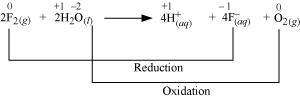20. Complete the following chemical reactions.
(i) PbS(g) + H2O2(aq) →
(ii) MnO4 aq +H2O(g) →
(iii) CaO(g)+H2O(g) →
(iv) AlCl3(g) + H2O(l) →
(v) Ca3N2(g) + H2O(l) →

Classify the above into
(a) Hydrolysis,
(b) Redox and
(c) Hydration reactions.

(i) PbS(g) + H2O2(aq) → PbSO4(s) + H2O(l)
H2Ois acting as an oxidizing agent in the reaction. Hence, it is a redox reaction

(ii) 2MnO4(aq)+ 5H2O → 6H+(aq) → 2Mn2+(aq) + 8H2O(l) + 5O2(g)

H2O2(aq) is acting as a reducing agent in the acidic medium, thereby oxidizing MnO4(aq) Hence, the given reaction is a redox reaction.

(iii) CaO(g) + H2O(g) → Ca(OH)2(aq)
The reactions in which a compound reacts with water to produce other compounds are called hydrolysis reactions. The given reaction is hydrolysis.

(iv) 2AlCl3(g) + 3H2O(l) → Al2O3(s) + 6HCl(aq)
The reactions in which a compound reacts with water to produce other compounds are called hydrolysis reactions. The given reaction represents hydrolysis of AlCl3.

(v) Ca3N2(s) + 6H2O(l) → 3Ca (OH)2(aq) +2NH3(g)
The reactions in which a compound reacts with water to produce other compounds are called hydrolysis reactions. The given reaction represents hydrolysis of Ca3N2.

21. Explain the structure of common form of ice.

Answer: Generally, ice is the crystalline form of water. It visibles in a hexagonal form if it is crystallized at atmospheric pressure. When the temperature is very low, it condenses to cubic form.
3 – D structure of ice: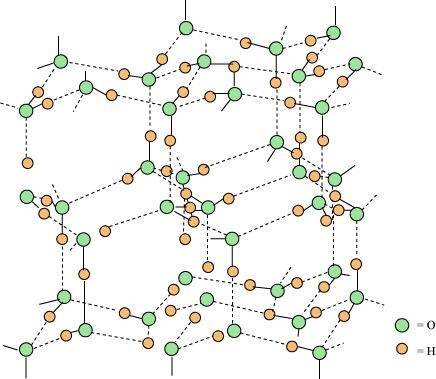It has hydrogen bonding and highly ordered structure. Each of the oxygen atom is surrounded tetrahedrally by 4 other oxygen atoms at a distance of 276 pm. The structure of ice also contains wide holes that can hold molecules of particular sizes.

22. What causes the permanent hardness and temporary hardness of water?
Answer: Due to the presence of soluble salts of magnesium and calcium in the form of chlorides in water, hardness remains permanent in water.
Due to the presence of soluble salts of calcium and magnesium in the form of hydrogen carbonates in water, hardness remains Temporary in water.

23. Explain the process and principle of softening of hard water by synthetic ion exchange resins.
Answer: The process of treating permanent hardness of water using synthetic resins generally based on exchange of anions and cations present in water by OH–  and H+ ions respectively.
Two types of synthetic resins are
1.Anion exchange resins
2.Cation exchange resins

Cation exchange resins are large organic molecules that contain the –SO3H group. Firstly the resin get changed into RNa by treating it with NaCl. This resin exchanges Na+ ions with Ca2+ ions and Mg2+ ions, thereby making the water soft.
2RNa + M2+(aq) → R2M(s) + 2Na+(aq)

There are cation exchange resins in H+ form. The resins exchange H+ ions for Na+, Ca2+, and Mg2+ ions.
2RH+M2+(aq) ⇌ MR2(s) + 2H+(aq)

Anion exchange resins exchange OH–  ions for anions like Cl , HCO3  and SO2−4 present in water.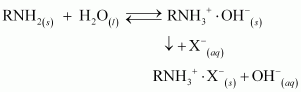During the whole process, first the water passes through the cation exchange process. The water which is obtained after this process is free from mineral cations and naturally acidic. This acidic water is then passed through the anion exchange process where OH– ions neutralize the H+ ions and de-ionize the water obtained.

24. Mention the chemical reaction which shows the amphoteric nature of water.
The amphoteric nature of water can be described on the basis of the following reactions:
1) Reaction with H2S
The reaction takes place as:
H2O(l) + H2S(aq) ⇌ H3O+(aq) + HS(aq)
In the forward reaction, H2O(l) accepts a proton from  H2S(aq). Therefore, it acts as a lewis base.

2) Reaction with NH3
The reaction takes place as:
H2O(l) + NH3(aq) ⇌ OH(aq) + NH+4(aq)
In the forward reaction, H2O(l) denotes its proton to NH3(aq). Therefore, it acts as lewis acid.

3) Self-ionization of water
2 water molecules reacts in this reaction as,
H2O(l) + H2O(l) ⇌ H3O+(aq) + OH(aq)

25. Hydrogen peroxide can act as oxidizing agent as well as reducing agent. Justify and write a chemical equation.
Answer: Hydrogen peroxide acts as an oxidizing agent as well as reducing agent in both alkaline medium and acidic medium.
The reaction which are involved in oxidizing actions are:
(i)  Mn2+ + H2O2 → Mn4+ + 2OH
(ii) 2Fe2+ + H2O→ 2Fe3+ + 2OH
(iii) 2Fe2+ + 2H+ + H2O2 → 2Fe3+ + 2H2O
(iv)  PbS + 4H2O2 → PbSO4+ 4H2O

The reaction which are involved in reduction actions are:
(i) I2 + H2O2 + 2OH → 2I+ 2H2O + O2
(ii) 2MnO4 + 3H2O2 → 2MnO2 + 3O2 + 2H2O + 2OH
(iii)  2MnO4 + 6H+ + 5H2O2 → 2Mn2+ + 8H2O + 5O2
(iv) HOCl + H2O2 → H3O+ + Cl + O2

26. Define ‘demineralised’ water and explain how it is obtained.
Demineralised water is free from all soluble mineral salts. It does not contain any anions or cations.
Demineralised water is obtained by passing water successively through a cation exchange (in the H+ form) and an anion exchange (in the OH− form) resin.
During the cation exchange process,H+ exchanges for Na+, Mg2+, Ca2+ , and other catons present in water.
2RH(s)+ M2+(aq) ⇄ MR2(s) + 2H+(aq) ……(1)
In the anion exchange process, OH− exchanges for anions such as CO2−3, SO2−4, Cl, HCO3 etc. present in water.
RNH2(s) + H2O(t) ⇄ RNH+3⋅OH(s)
RNH + 3OH(s) + X(aq) ⇄ RNH+3⋅ X(s)+OH−(aq)……..(2)
OH−ions liberated in reaction (2) neutralize  H+ ions liberated in reaction (1), thereby forming water.
H+(aq) + OH(aq)⟶H2O(l)

27. Is distilled water useful for drinking purposes? If not, explain how it can be used?
Answer: Water is very essential for our life. It consist of many dissolved nutrients that are required for us and also for plants and animals.  Demineralised water is free from all soluble minerals and so it cannot be used for drinking purpose.
After adding desired minerals in specific amounts which are required for growth,this water can be made useful.

28. Explain the uses of water in biological systems and biosphere.
Answer: Water is essential for all forms of life. It constitutes around 65% of the human body and 95% of plants. Water plays an important role in the biosphere owing to its high specific heat, thermal conductivity, surface tension, dipole moment, and dielectric constant.

The high heat of vapourization and heat of capacity of water helps in moderating the climate and body temperature of all living beings.

It acts as a carrier of various nutrients required by plants and animals for various metabolic reactions.

29. What properties of water make it useful as a solvent? What types of compound can it
(i) dissolve, and
(ii) hydrolyse ?

A high value of dipole moment and dielectric constants (78.39 C2 /Nm2 ) makes water a universal solvent. Water is able to dissolve most covalent and ionic compounds. Because of the ion-dipole interaction, Ionic compounds dissolve in water, whereas covalent compounds forms hydrogen bonding and dissolves in water. Water can hydrolyze
→ metallic and non-metallic oxides,
→, nitrides
→  phosphides,
→ carbides,
→ hydrides
and various other salts. During hydrolysis, H+ and OH–  ions of water interact with the reacting molecule.
Certain reactions are:
CaC2 + H2O → C2H2 + Ca(OH)2

CaO+H2O → Ca(OH)2

NaH+H2O → NaOH + H2

30. By knowing the properties of D2O and H2O, is it possible to use D2O for drinking purposes?
D2O is known as heavy water which acts as moderator (slows down the rate of reaction). Due to this peoperty, it cannot be used for drinking purpose because it slows down
(i) catabolic reaction and
(ii) anabolic reaction
That takes place in the body which leads to casualty.

31. Write the difference between hydration and hydrolysis.
Hydration:The addition of 1 or more molecules to a molecule or ion which results in formation of hydrated compounds is known as hydration.

E.g. CuSO4 + 5H2O→ CuSO4.5H2O

Hydrolysis: Chemical reaction in which hydroxide ions and hydrogen of water molecules react with a compound to form products is called hydrolysis.

E.g. NaH+H2O→NaOH+H2

32. From organic compounds, how can saline hydrides remove traces of water?
Answer: Naturally, saline hydrides are ionic. Saline hydrides reacts with water which results in the formation of metal hydroxide along with hydrogen gas liberation. It is represented as,
AH(s) + H2O(l) → AOH(aq) + H2(g)

When added to an organic solvent, they react with water present in it. Hydrogen escapes into the atmosphere leaving behind the metallic hydroxide. The dry organic solvent distills over.

33. What do you expect the nature of hydrides is, if formed by elements of atomic numbers 15, 19, 23 and 44 with dihydrogen? Compare their behaviour towards water.

The elements of atomic numbers 15, 19, 23, and 44 are phosphorus, potassium, vanadium, and ruthenium respectively.

1) Hydride of phosphorus
Hydride of nitrogen (PH3) is a covalent molecule. It is an electron-rich hydride owing to the presence of excess electrons as a lone pair on phosphorus.

2) Hydride of potassium
Dihydrogen forms an ionic hydride with potassium owing to the high electropositive nature of potassium. It is crystalline and non-volatile in nature.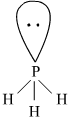3) Hydrides of Vanadium and Ruthenium
Both vanadium and ruthenium belong to the d–block of the periodic table. The metals of d–block form metallic or non–stoichiometric hydrides. Hydrides of vanadium and ruthenium are therefore, metallic in nature having a deficiency of hydrogen.

4) Behaviour of hydrides towards water
Potassium hydride reacts violently with water as :

KH(s)+ H2O(αq) ⟶ KOH(αq) + H2(g)
Phosphorus (PH3)  is covalent hydride and slightly soluble in water.
Hydrides of vanadium and Ruthenium do not react with water. Hence, the increasing order of reactivity of the hydrides is (V, Ru) H < NH3 < KH..

34. Do you expect different products in solution when aluminium (III) chloride and potassium chloride treated separately with
(i) alkaline water
(ii) acidified water, and
(iii) normal water
Write equations wherever necessary.

Potassium chloride (KCl) is the salt of a strong acid (HCl) and strong base (KOH). Hence, it is neutral in nature and does not undergo hydrolysis in normal water. It dissociates into ions as follows:
KCl(g) → K+(aq)+ Cl(aq)

In acidified and alkaline water, the ions do not react and remain as such. Aluminium (III) chloride is the salt of a strong acid (HCl) and weak base [Al(OH)3]. Hence, it undergoes hydrolysis in normal water.
AlCl3(g) + 3H2O(l) → Al (OH)3(s) + 3H+(aq) + 3Claq

In acidified water, H+ ions react with Al(OH)3 forming water and giving Al3+ ions. Hence, in acidified water, AlCl3 will exist as  Al3+(aq) and Cl(aq)

35. How does H2 O2 behave as a bleaching agent?
Hydrogen peroxide acts as a strong oxidizing agent both in basic and acidic media. When added to a cloth, it breaks the chemical bonds of the chromophores (colour producing agents). Hence, the visible light is not absorbed and the cloth gets whitened.

36. What do you understand by the terms:
(i) hydrogen economy
(ii) hydrogenation
(iii) ‘syngas’
(iv) water-gas shift reaction
(v) fuel-cell ?

→ Water-gas shift reaction

It is a reaction of carbon monoxide of syngas mixture with steam in the presence of a catalyst as: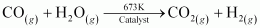This reaction is used to increase the yield of dihydrogen obtained from the coal gasification reaction as:
C(g)+H2O(g)→ CO(g) + H2(g)

→ Hydrogenation
The process of adding dihydrogen to another reactant is known as hydrogenation. It is used to reduce a compound in the presence of suitable catalyst.

E.g. Hydrogenation of vegetable oil using nickel as a catalyst gives edible fats such as ghee and vanaspathi.

→ Fuel cell
Fuel cells are devices for producing electricity from fuel in the presence of an electrolyte. Dihydrogen can be used as a fuel in these cells. It is preferred over other fuels because it is eco-friendly and releases greater energy per unit mass of fuel as compared to gasoline and other fuels.

→ Hydrogen economy
Dihydrogen releases more energy than petrol and is more eco–friendly. Hence, it can be used in fuel cells to generate electric power. Hydrogen economy is a technique of using dihydrogen in an efficient way. It involves transportation and storage of dihydrogen in the form of liquid or gas. It  is about the transmission of this energy in the form of dihydrogen.

→ ‘syngas’
Syngas is a mixture of carbon monoxide and dihydrogen. Since the mixture of the two gases is used for the synthesis of methanol, it is called syngas, synthesis gas, or water gas.
Syngas is produced on the action of steam with hydrocarbons or coke at a high temperature in the presence of a catalyst.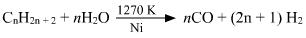For example :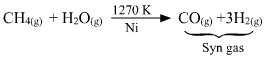## Conclusions for NCERT SOLUTIONS FOR CLASS 11 CHEMISTRY CHAPTER 9- HYDROGEN

An academic team of knowledgeable members of SWC has produced and published the NCERT Solutions for class 11’s Chemistry chapter for your use as a reference. You can get answers to all of the chapters of the NCERT Chemistry class 11 here at SWC. Please make use of the following NCERT answers that were created by SWC as a reference for this chapter. In addition to that, study the chapter’s theory before attempting to solve the NCERT problems.